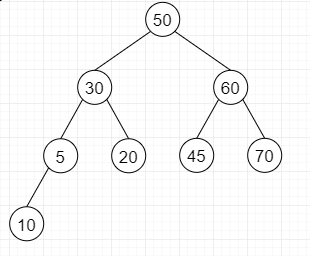# Find the largest Complete Subtree in a given Binary Tree in Python

Suppose we have a Binary Tree; we have to find the size of maximum complete sub-tree in this Binary Tree. As we know a complete binary tree is a Binary Tree if all levels are completely filled without possibly the final level and the final level has all keys as left as possible.

So, if the input is likethen the output will be 4 as size and inorder traversal will be 10, 45, 60, 70,

To solve this, we will follow these steps −

• Define return type with few parameters like isComplete, isPerfect, these are initially false, then size and rootTree, size is initially 0 and rootTree is null.
• ret_type := returnType
• if root is null, then
• ret_type.isPerfect := True
• ret_type.isComplete := True
• ret_type.size := 0
• ret_type.rootTree := None
• return ret_type
• left_tree := checkCompleteness(root.left)
• right_tree := checkCompleteness(root.right)
• if (left_tree.isPerfect is True and right_tree.isComplete is True and height of left and right tree are same, then
• ret_type.isComplete := True
• ret_type.isPerfect := right_tree.isPerfect
• ret_type.size := left_tree.size + right_tree.size + 1
• ret_type.rootTree := root
• return ret_type
• if (left_tree.isComplete is True and right_tree.isPerfect is True and height of left and right tree are same, then
• ret_type.isComplete := True
• ret_type.isPerfect := False
• ret_type.size := left_tree.size + right_tree.size + 1
• ret_type.rootTree := root
• return ret_type
• ret_type.isPerfect := False
• ret_type.isComplete := False
• ret_type.size := maximum of left_tree.size, right_tree.size
• if left_tree.size > right_tree.size, then
• ret_type.rootTree := left_tree.rootTree
• otherwise,
• ret_type.rootTree := right_tree.rootTree
• return ret_type

## Python

Let us see the following implementation to get better understanding −

import math
class TreeNode:
def __init__(self, data, left = None, right = None):
self.data = data
self.left = left
self.right = right
class returnType :
def __init__(self):
self.isPerfect = None
self.isComplete = None
self.size = 0
self.rootTree = None
def getHeight(size):
return int(math.ceil(math.log(size + 1)/math.log(2)))
def checkCompleteness(root) :
ret_type = returnType()
if (root == None):
ret_type.isPerfect = True
ret_type.isComplete = True
ret_type.size = 0
ret_type.rootTree = None
return ret_type
left_tree = checkCompleteness(root.left)
right_tree = checkCompleteness(root.right)
if (left_tree.isPerfect == True and right_tree.isComplete == True and getHeight(left_tree.size) == getHeight(right_tree.size)) :
ret_type.isComplete = True
ret_type.isPerfect = right_tree.isPerfect
ret_type.size = left_tree.size + right_tree.size + 1
ret_type.rootTree = root
return ret_type
if (left_tree.isComplete == True and right_tree.isPerfect == True and getHeight(left_tree.size) == getHeight(right_tree.size) + 1):
ret_type.isComplete = True
ret_type.isPerfect = False
ret_type.size = left_tree.size + right_tree.size + 1
ret_type.rootTree = root
return ret_type
ret_type.isPerfect = False
ret_type.isComplete = False
ret_type.size =max(left_tree.size, right_tree.size)
if(left_tree.size > right_tree.size ):
ret_type.rootTree = left_tree.rootTree
else:
ret_type.rootTree = right_tree.rootTree
return ret_type
def print_tree(root):
if root is not None:
print_tree(root.left)
print(root.data, end = ', ')
print_tree(root.right)
root = TreeNode(50)
root.left = TreeNode(30)
root.right = TreeNode(60)
root.left.left = TreeNode(5)
root.left.right = TreeNode(20)
root.right.left = TreeNode(45)
root.right.right = TreeNode(70)
root.right.left.left = TreeNode(10)
ans = checkCompleteness(root)
print( "Size:" , ans.size )
print("Inorder Traversal: ", end = '')
print_tree(ans.rootTree)

## Input

root = TreeNode(50)
root.left = TreeNode(30)
root.right = TreeNode(60)
root.left.left = TreeNode(5)
root.left.right = TreeNode(20)
root.right.left = TreeNode(45)
root.right.right = TreeNode(70)
root.right.left.left = TreeNode(10)

## Output:

Size: 4
Inorder Traversal: 10, 45, 60, 70,